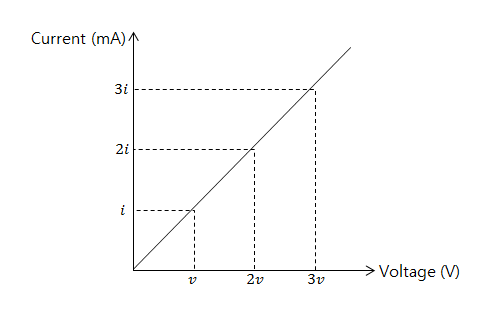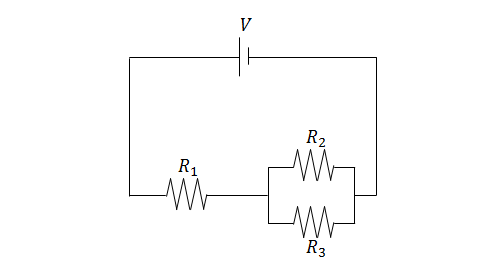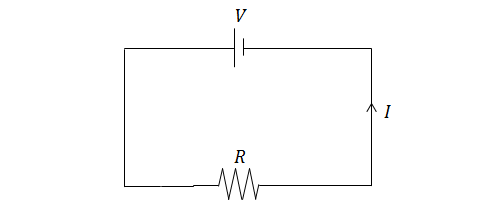Electricity and Magnetism

# Ohm's lawThe above graph shows the current through a nichrome wire according to the voltage applied. If $$i=\si{200\ \milli\ampere}$$ and $$v=\si{125\ \volt}$$,) what is the resistance (in $$\Omega$$) of the nichrome wire?

It is known that the amount of current needed to flow through a human being in order to cause them to have ventricular fibrillation (heart attack) is about 100 mA.

What is the minimum voltage (in volts) that will cause a person who has a body resistance of $$1723\ \Omega$$ to have ventricular fibrillation?

If a current $$I=0.9\text{ A}$$ flows through a resistor $$R=7\ \Omega$$ of length $$\Delta d=5\$$ m, what is the electric field strength inside the resistor?In the circuit above, $$V=5\text{ V},$$ $$R_1=1\ \Omega,$$ $$R_2=6\ \Omega,$$ and $$R_3=12\ \Omega.$$ Find the current through $$R_1.$$If $$I=1\text{ A}$$ and $$R=8\ \Omega,$$ what is the voltage of the battery?

×